# Exercise E10.6 Circles NCERT Solutions Class 9

## Chapter 10 Ex.10.6 Question 1

Prove that the line of centres of two intersecting circles subtends equal angles at the two points of intersection.

### Solution

What is known?

Two intersecting circles.

What is unknown?

To prove that the line of centres of two intersecting circles subtends equal angles at the two points of intersection

Reasoning:

Using Side-Side-Side (SSS criteria) and Corresponding parts of congruent triangles (CPCT) we prove the statement.

Steps:

Draw $$2$$ intersecting circles with centres $${O}$$ and $${O’}$$ respectively. Join these $$2$$ centres.

Let the points of intersection be $${A}$$ and $${B.}$$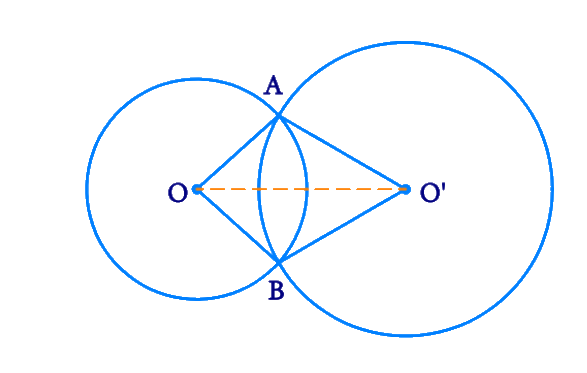We need to prove that

\begin {align}\angle {OAO}^{\prime}=\angle {OBO}^{\prime} \end {align}

Consider \begin {align}\Delta {OAO}^{\prime} \end {align} and \begin {align}\Delta {OBO}^{\prime} \end {align}

\begin{align}{OA}&={OB}\\ \text { (Radii of circle}&\text{ with centre O)}\\\\ {O}^{\prime} {A}&={O}^{\prime } {B} \\ \text { (Radii of circle} & \text {with centre O^\prime )} \\\\{OO}^{\prime}&={O} {O}^{\prime} \\&\text { (Common) }\end{align}

Therefore by SSS criteria, \begin {align}\Delta {O} {AO}^{\prime} \end {align} and \begin {align}\Delta {O} {BO}^{\prime} \end {align} are congruent to each other.

\begin {align}{\rm{By\,\, CPCT}} , \;\angle {OAO}^{\prime}=\angle {OBO}^{\prime} \end {align}

Hence it is proved that the line of centres of two intersecting circles subtends equal angles at the two points of intersection.

## Chapter 10 Ex.10.6 Question 2

Two chords \begin {align}{AB}\end {align} and \begin {align}{CD}\end {align} of lengths \begin {align}\text{5 cm}\end {align} and \begin {align}\text{11 cm}\end {align} respectively of a circle are parallel to each other and are on opposite sides of its centre. If the distance between \begin {align}{AB}\end {align} and \begin {align}{CD}\end {align} is \begin {align}\text{6 cm,}\end {align} find the radius of the circle.

### Solution

What is known?

Two parallel chords of lengths $$5\rm{ cm}$$ and $$11\rm{ cm}$$ and the distance between parallel chords is $$6\rm{ cm.}$$

#### What is unknown?

Radius of the circle.

Reasoning:

The perpendicular drawn from the centre of the circle to the chords, bisects it.

Pythagoras theorem states that

(Side $$1)^{2}$$ $$+$$ (Side $$2)^{2} =$$ (Hypotenuse )$$^{2}$$

Steps:

Draw $$2$$ parallel chords \begin {align}{AB}\end {align} and \begin {align}{CD}\end {align} of lengths \begin {align}\text{5 cm}\end {align} and \begin {align}\text{11 cm.}\end {align} Let the centre of the circle be \begin {align}{O. }\end {align} Join one end of each chord to the centre. Draw $$2$$ perpendiculars \begin {align}{OM}\end {align} and \begin {align}{ON}\end {align} to \begin {align}{AB}\end {align} and \begin {align}{CD }\end {align} respectively which bisects the chords.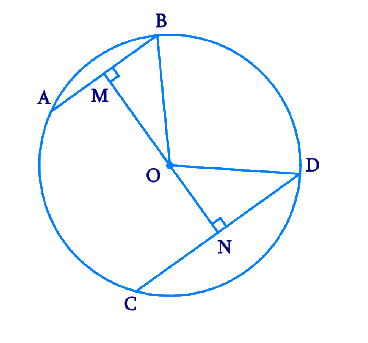\begin {align}{AB}&=5 \mathrm{cm} \\ {CD}&=11 \mathrm{cm} \\ {MB}&=2.5 \mathrm{cm} \\ {ND}&=5.5 \mathrm{cm}\end{align}

Let  $$OM = x \,\,\rm{cm}$$

and  $$ON = 6 – x\,\,\rm{cm}$$

Consider \begin{align}\Delta {OMB}\end {align}

By Pythagoras theorem,

\begin{align} {OM}^{2}+{MB}^{2} &={OB}^{2} \\ x^{2}+2.5^{2} &={OB}^{2} \\ x^{2}+6.25 &={OB}^{2} \ldots .(1) \end{align}

Consider \begin{align}\Delta {OND}\end {align}

By Pythagoras theorem,

\begin{align}{ON}^{2}+{ND}^{2}&={OD}^{2} \\ (6-x)^{2}+5.5^{2}&={OD}^{2} \\ \begin{Bmatrix} 36+x^{2} \\ -12 x+30.25 \end{Bmatrix} &={OD}^{2} \\ \begin{Bmatrix} x^{2}-12 x \\ +66.25 \end{Bmatrix} &={OD}^{2} \ldots .(2)\end{align}

\begin{align} {OB}\end{align} and \begin{align} {OD}\end{align} are the radii of the circle. Therefore \begin{align} {OB = OD.}\end{align}

Equating ($$1$$) and ($$2$$) we get,

\begin{align}x^{2}+6.25&=x^{2}-12 x+66.25 \\ 12 x&=60 \\ x&=5\end{align}

Substituting the value of \begin{align} x\end{align} in ($$1$$) or ($$2$$) we get the radius of circle $$= 5.59 \rm cm.$$

## Chapter 10 Ex.10.6 Question 3

The lengths of two parallel chords of a circle are \begin{align} \text{6 cm}\end{align} and \begin{align} \text{8 cm.}\end{align} If the smaller chord is at distance \begin{align} \text{4 cm}\end{align} from the centre, what is the distance of the other chord from the centre?

### Solution

What is known?

Two parallel chords of lengths $$6\,\rm{ cm}$$ and $$8\,\rm{ cm}$$ and distance of smaller chord from centre is $$4\,\rm{cm.}$$

What is unknown?

Radius of the circle and distance of one chord from centre.

Reasoning:

The perpendicular drawn from the centre of the circle to the chords, bisects it.

Pythagoras theorem states that

(Side $$1)^{2}$$ $$+$$ (Side $$2)^{2} =$$ (Hypotenuse )$$^{2}$$

Steps:

Draw $$2$$ parallel chords \begin{align} {AB}\end{align} and \begin{align} {CD}\end{align} of lengths \begin{align} \text{6 cm}\end{align} and \begin{align} \text{8 cm.}\end{align} Let the centre of the circle be \begin{align} {O.}\end{align} Join one end of each chord to the centre. Draw $$2$$ perpendiculars \begin{align} {OM}\end{align} and \begin{align} {ON}\end{align} to \begin{align} {AB}\end{align} and \begin{align} {CD }\end{align} respectively which bisects the chords.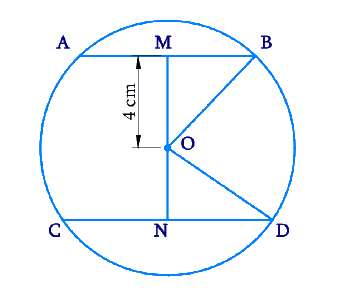\begin{align} {AB} &=6 \, \rm{cm} \\ {CD} &=8 \, \rm{cm} \\{MB} &=3 \, \rm{cm} \\ {ND} &=4 \,\rm{cm} \end{align}

Given $$OM = \text{4 cm }$$

and let $${ON =} x\, \rm{cm}$$

Consider \begin{align} \Delta {OMB}\end{align}

By Pythagoras theorem,

\begin{align}{OM}^{2}+{MB}^{2}&={OB}^{2} \\ 4^{2}+3^{2}&={OB}^{2} \\ 16+9&={OB}^{2} \\ {OB}^{2}&=25 \\ {OB}&=5 \,\mathrm{cm}\end{align}

\begin{align}{OB}\end{align} and \begin{align}{OD}\end{align} are the radii of the circle.

Therefore $$OD = OB =\text{ 5 cm.}$$

Consider \begin{align}\Delta{OND}\end{align}

By Pythagoras theorem,

\begin{align}{ON}^{2}+{ND}^{2}&={OD}^{2} \\ x^{2}+4^{2}&=5^{2} \\ x^{2}&=25-16 \\ x^{2}&=9 \\ x&=3 \, \mathrm{cm}\end{align}

The distance of the chord \begin{align}{CD}\end{align} from the centre is \begin{align}\text{3 cm.}\end{align}

## Chapter 10 Ex.10.6 Question 4

Let the vertex of an angle \begin{align}{ABC}\end{align} be located outside a circle and let the sides of the angle intersect equal chords \begin{align}{AD}\end{align} and \begin{align}{CE}\end{align} with the circle. Prove that \begin{align} \angle {ABC}\end{align} is equal to half the difference of the angles subtended by the chords \begin{align}{AC}\end{align} and \begin{align}{DE}\end{align} at the centre.

### Solution

What is known?

Point $$B$$ lies outside the circle also $$AD$$ and $$CE$$ are equal chords.

What is unknown?

To prove that \begin{align} \angle {ABC}\end{align} is equal to half the difference of the angles subtended by the chords \begin{align} {AC}\end{align} and \begin{align}{DE}\end{align} at the centre means \begin{align}\angle ABC = \frac{1}{2}\left( {\angle DOE - \angle AOC} \right)\end{align}

Reasoning:

• The angle subtended by an arc at the centre is double the angle subtended by it at any point on the remaining part of the circle.
• A quadrilateral \begin{align}{ACED}\end{align} is called cyclic if all the four vertices of it lie on a circle.
• The sum of either pair of opposite angles of a cyclic quadrilateral is $$180^\circ.$$
• Using Side-Side-Side (SSS criteria) and Corresponding parts of congruent triangles (CPCT) we prove the statement.

Steps: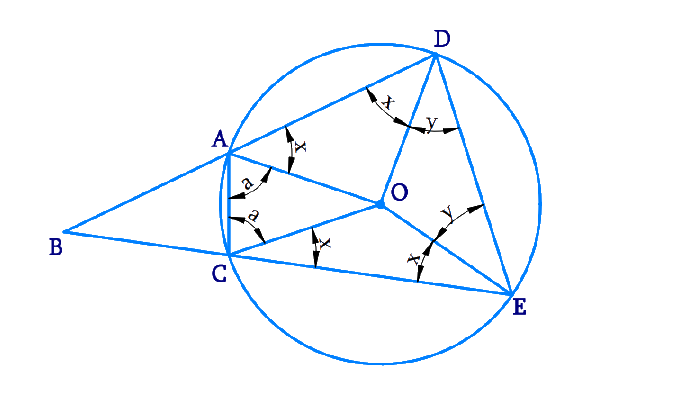Consider $$\Delta {AOD}$$ and $$\Delta {COE,}$$

\begin{align}{OA}&={OC} \qquad {\text { (Radii of the circle) }} \\{OD}&={OE} \qquad {\text { (Radii of the circle) }} \\ {AD}&={CE} \qquad {\text { (Given) }}\end{align}

$$∴ \Delta {AOD} \cong \Delta {COE}$$ (SSS congruence rule )

$$\angle {OAD}=\angle {OCE}$$ (By CPCT )  ....($$1$$

$$\angle {ODA}=\angle {OEC}$$ (By CPCT )  .....($$2$$

Also,

$$\!\angle {OAD}\!=\!\angle {ODA}$$(As $$OA=OD$$)...($$3$$)

From Equations ($$1$$), ($$2$$), and ($$3$$), we obtain

$\!\! \angle OAD \! = \!\angle OCE = \!\!\angle {ODA}= \!\! \angle {OEC}$

\begin {align} \angle {OAD} & =\angle {OCE} \\ &=\angle {ODA}\\ &=\angle {OEC}\\ &=x \end {align}

In \begin {align} \Delta {OAC,} \end {align}

\begin{align} {OA} &={OC} \\ ∴ {OCA} &=\angle {OAC}(\text { Let } a) \end{align}

In \begin {align} \Delta {ODE,} \end {align}

\begin{align} \rm{OD} &=\rm{OE} \\ \angle \rm{OED} &=\angle \rm{ODE}\text{ (Let y)} \end{align}

\begin{align}\rm{ADEC}\end{align} is a cyclic quadrilateral.

$$∴ \angle {CAD}+\angle {DEC}=180^{\circ}$$ (Opposite angles are supplementary)

\begin{align} x+a+x+y&=180^{\circ} \\ \!2 x+a+y&=180^{\circ} \\ y \!&=\!180^{\circ}-2 x \! -a ...\! (4) \end{align}

However, $$\angle {DOE}=180^{\circ}-2 {y}$$

And, \begin {align}\angle{AOC}=180^{\circ}-2 {a} \end {align}

\begin{align} & \angle {DOE}-\angle {AOC} \\&=2a-2y \\ & =2 a-2\left(180^{\circ}-2 x-a\right) \\ &=4 a+4 x-360^{\circ} \ldots \ldots (5) \end{align}

$$\angle {BAC}+\angle {CAD}=180^{\circ}$$(Linear pair )

\begin{align} ∴ \angle {BAC}&=180^{\circ}-\angle{CAD} \\ &=180^{\circ}-(a+x)\end{align}

Similarly, \begin {align} \angle {ACB}=180^{\circ}-(a+x) \end {align}

In \begin {align} \Delta {ABC,} \end {align}

$$\angle {ABC}+\angle {BAC}+\angle {ACB}=180^{\circ}$$

(Angle sum property of a triangle)

\begin {align}\angle {ABC} &=180^{\circ}-\angle {BAC}-\angle {ACB} \\ &= \begin{Bmatrix} 180^{\circ} \\ -\left(180^{\circ}-a-x\right) \\ -\left(180^{\circ}-a-x\right) \end{Bmatrix} \\ &=2 a+2 x-180^{\circ} \\ &=\frac{1}{2}\left[4 a+4 x-360^{\circ}\right]\end {align}

Using Equation ($$5$$)

\begin {align}\angle {ABC}=\frac{1}{2}[\angle {DOE}-\angle {AOC}] \end {align}

Hence it is proved that \begin{align} \angle {ABC}\end{align} is equal to half the difference of the angles subtended by the chords \begin{align}{AC}\end{align} and \begin{align}{DE}\end{align} at the centre.

## Chapter 10 Ex.10.6 Question 5

Prove that the circle drawn with any side of a rhombus as diameter, passes through the point of intersection of its diagonals.

### Solution

What is known?

Diameter of the circle is the length of one side of rhombus.

What is unknown?

Proof of  the circle passes through the point of intersection of rhombus diagonals.

Reasoning:

The diagonals of rhombus intersect at \begin{align}90^\circ.\end{align}

Steps: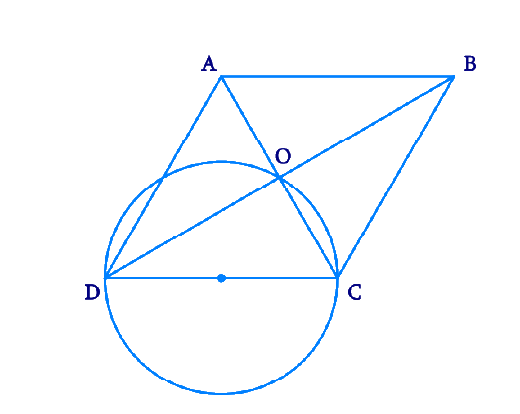Let \begin{align}{ABCD}\end{align} be a rhombus in which diagonals are intersecting at point \begin{align}{O}\end{align} and a circle is drawn while taking side \begin{align}{CD}\end{align} as its diameter. We know that a diameter subtends \begin{align}90^\circ\end{align} on the arc.

\begin{align}∴{COD }= 90^\circ\end{align}

Also, in rhombus, the diagonals intersect each other at \begin{align}90^\circ.\end{align}

\begin {align} \angle {AOB} & =\angle {BOC}\\ &=\angle {COD}\\ & =\angle {DOA} \\ & =90^{\circ}\end {align}

Clearly, point \begin {align}{O} \end {align} has to lie on the circle.

## Chapter 10 Ex.10.6 Question 6

\begin {align}{ABCD} \end {align} is a parallelogram. The circle through \begin {align}{A, B} \end {align} and \begin {align}{C} \end {align} intersect \begin {align}{CD} \end {align} (produced if necessary) at \begin {align}{E} \end {align}. Prove that \begin {align}{AE = AD.} \end {align}

### Solution

What is known?

\begin {align}{ABCD} \end {align} is a parallelogram.

What is unknown?

Proof that \begin {align}{AE = AD} \end {align}

Reasoning:

• A quadrilateral \begin {align}{ABCD} \end {align} is called cyclic if all the four vertices of it lie on a circle.
• The sum of either pair of opposite angles of a cyclic quadrilateral is $$180^{\circ}.$$
• Opposite angles in a parallelogram are equal.

Steps: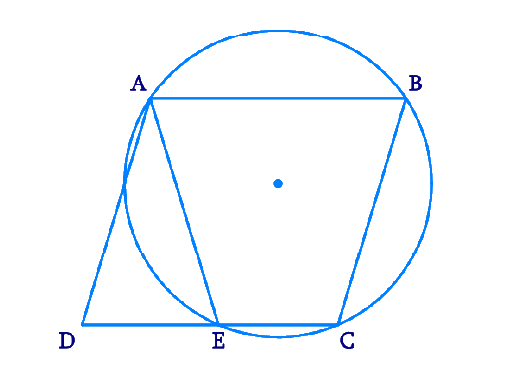We can see that \begin {align}{ABCE} \end {align} is a cyclic quadrilateral.

We know that in a cyclic quadrilateral, the sum of the opposite angles is $$180^{\circ.}$$

\begin{align}\angle {AEC}+\angle {CBA}&=180^{\circ} \\ \angle {AEC} +\angle {AED}&=180^{\circ} (\text { Linear pair })\end{align}

$$\angle {AED}=\angle {CBA} \ldots \text { (1) }$$

We know that in a parallelogram, opposite angles are equal.

\begin {align} \angle {ADE}=\angle{CBA}\quad \dots(2) \end {align}

From ($$1$$) and ($$2$$),

\begin{align} \angle {AED}=\angle{ADE}\\{AD}={AE}\qquad \end{align}

(sides opposite to equal Angles of a triangle are equal).

## Chapter 10 Ex.10.6 Question 7

\begin {align}{AC}\end {align} and \begin {align} {BD}\end {align} are chords of a circle which bisect each other. Prove that

(i) \begin {align} {AC}\end {align} and \begin {align} {BD}\end {align} are diameters,

(ii) \begin {align} {ABCD}\end {align} is a rectangle.

### Solution

What is known?

\begin {align} {AC}\end {align} and \begin {align}{BD}\end {align} are $$2$$ chords of a circle which bisect each other.

What is unknown?

Proof that \begin {align} {AC}\end {align} and \begin {align} {BD}\end {align} are diameters and \begin {align} {ABCD}\end {align} is a rectangle.

Reasoning:

• A quadrilateral \begin {align} {ABCD}\end {align} is called cyclic if all the four vertices of it lie on a circle.
• The sum of either pair of opposite angles of a cyclic quadrilateral is $$180^{\circ}.$$
• Opposite angles in a parallelogram are equal.
• Using Side-Angle-Side (SAS criteria) and Corresponding parts of congruent triangles (CPCT) we prove the statement.

Steps: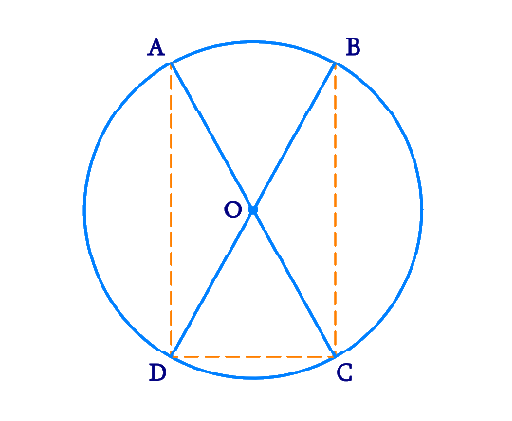Let \begin {align} {AC}\end {align} and \begin {align} {BD}\end {align} be $$2$$ chords intersecting at \begin {align} {O.}\end {align}

In \begin {align} \Delta {AOB}\end {align} and \begin {align} \Delta {COD,}\end {align}

\begin{align}{OA}&={OC} \quad (\text { Given }) \\ {OB}&={OD} \quad \text { (Given) } \\ \angle {AOB}&=\angle {COD} \\ \text {(Vertically } & \text{opposite} \text{ angles }) \end{align}

$$∴ \Delta {AOB} \cong \Delta {COD}$$ (SAS congruence rule)

$${AB} ={CD}$$ (By CPCT)

Similarly, it can be proved that \begin{align} \Delta {AOD} \cong \Delta {COB} \end{align}

\begin{align} ∴ {AD}={CB} \qquad (\text{By CPCT}) \end{align}

Since in quadrilateral \begin{align} {ABCD,} \end{align} opposite sides are equal in length, \begin{align} {ABCD} \end{align} is a parallelogram.

We know that opposite angles of a parallelogram are equal.

\begin{align} ∴ \angle {A}=\angle {C} \end{align}

However,

\begin{align} \angle {A} \!+ \! \angle {C} \!&=\!180^{\circ} \\{(ABCD} &\text { is a cyclic } \text{ quadrilateral) } \\\angle {A}+\angle {A} &=180^{\circ} \\ 2 \angle {A}&=180^{\circ} \\ ∴ \angle {A}&=90^{\circ}\end{align}

As \begin{align}{ABCD}\end{align} is a parallelogram and one of its interior angles is $$90^{\circ,}$$ therefore, it is a rectangle.

\begin{align}\angle {A}\end{align} is the angle subtended by chord \begin{align}\angle {BD.}\end{align}

And as .\begin{align}\angle {A} = 90^{\circ}, \end{align} therefore, \begin{align} {BD}\end{align} should be the diameter of the circle. Similarly, \begin{align} {AC}\end{align} is the diameter of the circle.

## Chapter 10 Ex.10.6 Question 8

Bisectors of angles \begin{align} {A, B}\end{align} and \begin{align} {C}\end{align} of a triangle \begin{align} {ABC}\end{align} intersect its circumcircle at \begin{align} {D, E}\end{align} and \begin{align}{F}\end{align} respectively. Prove that the angles of the triangle \begin{align} {DEF}\end{align} are \begin {align} 90^{0}-\frac{1}{2} {A}, \,90^{\circ}-\frac{1}{2} {B},\, 90^{\circ}-\frac{1}{2} {C}\end {align}

### Solution

What is known?

Bisectors of angles \begin {align} {A, B} \end {align} and \begin {align} {C} \end {align} of a triangle \begin {align} {ABC} \end {align} intersect its circumcircle at \begin {align} {D, E} \end {align} and \begin {align} {F } \end {align} respectively.

What is unknown?

Proof that the angles of the triangle \begin {align} \text{DEF} \end {align} are

$90^{0}-\frac{1}{2} {A}, \;\; 90^{\circ}-\frac{1}{2} {B}, \;\; 90^{\circ}-\frac{1}{2} {C}$

Reasoning:

Angles in the same segment are equal.

Steps: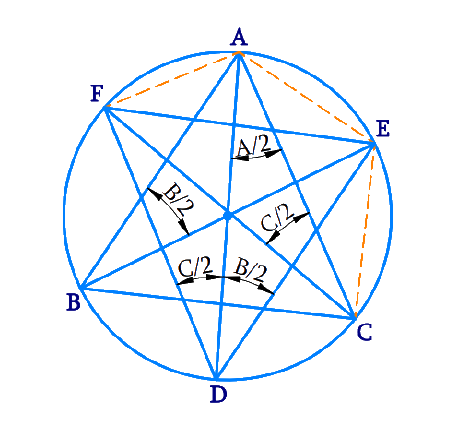It is given that \begin {align} {BE}\end {align} is the bisector of \begin {align} \angle {B.}\end {align}

\begin{align} \angle {ABE}=\frac{\angle {B}}{2} \end{align}

However,

\begin{align} \angle {ADE}&=\angle {ABE} \\\text { (Angles in} &\text{ the same} \text{ segment }\\& \text{ for chord AE })\\ \angle {ADE}&=\frac{\angle {B}}{2}\end{align}

Similarly,

\begin{align}\angle {ADF}&=\angle {ACF}\\&=\frac{\angle {C}}{2}\\\text { (Angles in} & \text{ the same} \text{ segment } \\ &\text{ for chord AF })\end {align}

\begin{align} \angle {D} &=\angle {ADE}+\angle {ADF} \\ &=\frac{\angle {B}}{2}+\frac{\angle {C}}{2} \\ &=\frac{1}{2}(\angle {B}+\angle {C}) \\ &=\frac{1}{2}\left(180^{\circ}-\angle {A}\right) \end{align}

Similarly it can be proved for

\begin{align} \angle {E} &=\frac{1}{2}\left(180^{\circ}-\angle {B}\right) \\ \angle {F} &=\frac{1}{2}\left(180^{\circ}-\angle {C}\right) \end{align}

## Chapter 10 Ex.10.6 Question 9

Two congruent circles intersect each other at points \begin{align}{A} \end {align} and \begin{align}{B.}\end {align} Through \begin{align}{A} \end {align} any line segment \begin{align}{PAQ} \end {align} is drawn so that \begin{align}{P, Q} \end {align} lie on the two circles. Prove that \begin{align}{BP = BQ.} \end {align}

### Solution

What is known?

Two congruent circles intersect each other at points \begin{align}{A} \end {align} and \begin{align}{B.} \end {align}

To prove:

\begin{align}{BP = BQ.} \end {align}

Reasoning:

The angle subtended by an arc at the centre is double the angle subtended by it at any point on the remaining part of the circle.

Steps: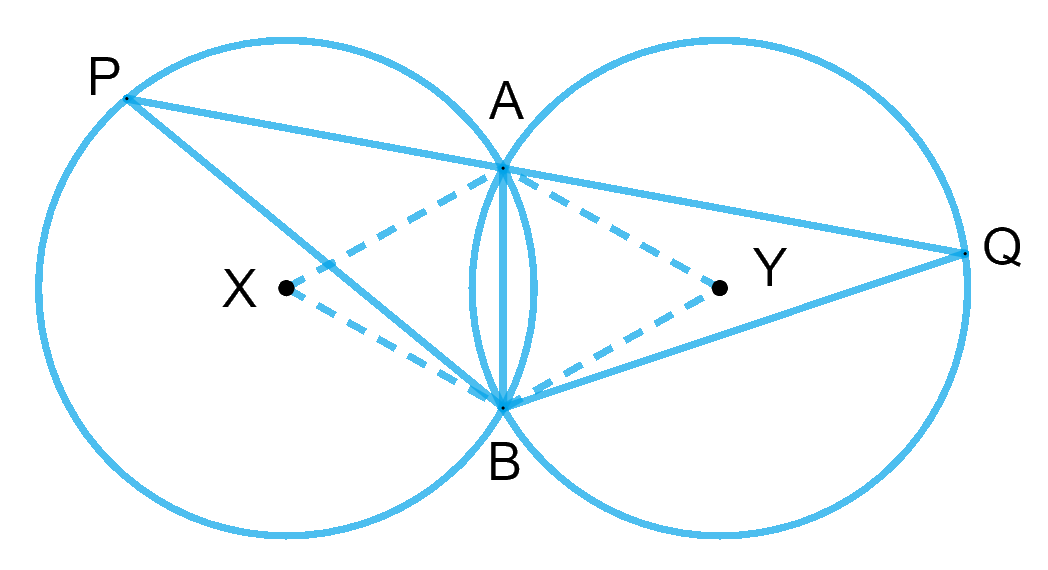\begin{align}{AB} \end {align} is the common chord to both circles. Let us join A and B to the centres $$X$$ and $$Y$$ of the respective circles.

As we know that, the angle subtended by an arc at the centre is double the angle subtended by it at any point on the remaining part of the circle.

Therefore,

\begin{align} \angle AXB &= 2\angle APB \\ \angle AYB &= 2\angle AQB \\ \end{align}

Since the circles are congruent and $$AB$$ is a common chord.

Hence, $$\Delta AXB \cong \Delta AYB$$ by SSS criteria of congruency.

$$\angle AXB = \angle AYB$$ by CPCT

Therefore, $$\angle APB = \angle AQB$$

Now, consider the $$ΔBPQ,$$

$\angle QPB = \angle PQB$

This implies that $$ΔBPQ$$ is an isosceles triangle as base angles are equal.

Therefore, we get $$BP = BQ.$$

## Chapter 10 Ex.10.6 Question 10

In any triangle \begin {align} {ABC,} \end {align} if the angle bisector of $$\angle {A}$$ and perpendicular bisector of $${BC}$$ intersect, prove that they intersect on the circumcircle of the triangle $${ABC.}$$

### Solution

What is known?

Bisectors of angle $$\angle {A}$$ and perpendicular bisector of $${BC}$$  intersect.

What is unknown?

Proof of the intersecting points is circumcircle.

Reasoning:

• Angles in the same segment are equal.
• The angle subtended by an arc at the centre is double the angle subtended by it at any point on the remaining part of the circle.

Steps: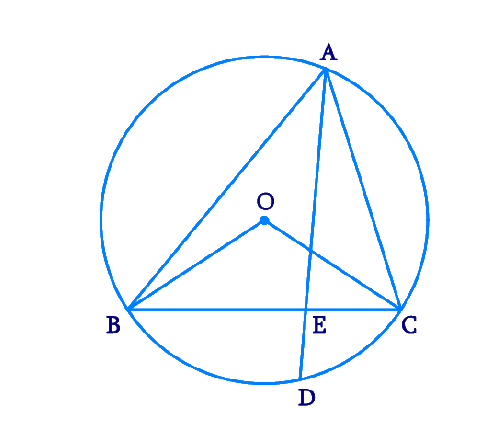Let perpendicular bisector of side $${BC}$$ and angle bisector of $$\angle {A}$$ meet at point $${D.}$$ Let the perpendicular bisector of side $${BC}$$ intersect it at $${E.}$$

Perpendicular bisector of side $${BC}$$ will pass through circumcentre $${O}$$ of the circle.

$$\angle {BOC}$$ and $$\angle {BAC}$$ are the angles subtended by arc $${BC}$$ at the centre and a point $${A}$$ on the remaining part of the circle respectively. We also know that the angle subtended by an arc at the centre is double the angle subtended by it at any point on the remaining part of the circle.

\begin {align} \angle {BOC}=2 \angle {BAC}=2 \angle {A} \quad \ldots(1) \end {align}

Consider $$\angle {BOE}$$ and $$\angle {COE,}$$

\begin{align}{OE}&={OE} \quad (\text { Common }) \\ {OB}&={OC} \quad \begin{pmatrix}\! \text { Radii of} \\ \text{same circle }\!\!\end{pmatrix} \\ \angle {OEB}&=\angle {OEC} \\( \text {Each } 90^{\circ} \text { as } &{OD} \text{ perpendicular }{BC} )\end{align}

$$\therefore \Delta {BOE} \cong \angle {COE}$$ (RHS congruence rule )

$$\angle {BOE} =\angle {COE}$$ (By  CPCT) ....($$2$$)

However, \begin {align} \angle {BOE}+\angle {COE}=\angle {BOC} \end {align}

\begin{align} \;\therefore \angle {BOE}+\angle {BOE}&=2 \angle {A} \\ [\text {Using } \text{Equations } &(1) \text { and }(2)] \\ 2 \angle {BOE}&=2 \angle {A} \\ \angle {BOE}&=\angle {A}\\\angle{BOE}&=\angle {COE}\\&=\angle {A} \end{align}

The perpendicular bisector of side \begin{align}\text{BC} \end{align} and angle bisector of \begin{align}\angle {A} \end{align} meet at point \begin{align}{D.} \end{align}

\begin {align} \therefore \angle {BOD}=\angle {BOE}=\angle {A} \ldots(3) \end {align}

Since \begin{align}\angle {AD} \end{align} is the bisector of angle \begin {align} \angle {A,} \end {align}

\begin{align}\angle {BAD}&=\text { Half of angle } {A}. \\ \therefore 2 \angle {BAD}&=\angle {A} \qquad \ldots \text { (4) }\end{align}

From Equations ($$3$$) and ($$4$$), we obtain

\begin{align} \angle {BOD}=2 \angle {BAD} \end {align}

This can be possible only when point \begin{align}{BD}\end {align} will be a chord of the circle. For this, the point \begin{align}{D}\end {align} lies on the circumcircle.

Therefore, the perpendicular bisector of side \begin{align}{BC}\end {align} and the angle bisector of \begin{align} \angle {A}\end {align} meet on the circumcircle of triangle \begin{align}{ABC.}\end {align}

Instant doubt clearing with Cuemath Advanced Math Program